# NCERT Solutions for Class 7 Maths Ch 15 Visualising Solid Shapes| PDF Download

Chapter 15 Visualising Solid Shapes NCERT Solutions for Class 7 Maths is available here that will be useful in passing exams with flying colours and guide student in a better way. Here, you will be also able to download PDF of Chapter 15 Visualising Solid Shapes NCERT Solutions through which one can easily understand the basic concepts given in the chapter. It will provide opportunities to learn new things for a student and improve studying habits.

These NCERT Solutions are prepared as per the accordance of latest CBSE guidelines so you can score maximum marks. It is very necessary to grasp the key concepts of the chapter in order to obtain good marks in the exams.Exercise 15.1

1. Identify the nets which can be used to make cubes (cut out copies of the nets and try it):Cube’s nets are (ii), (iii), (iv) and (vi).

2. Dice are cubes with dots on each face. Opposite faces of a die always have a total of seven dots on them.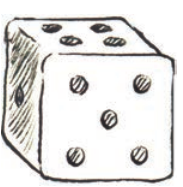Here are two nets to make dice (cubes); the numbers inserted in each square indicate the number of dots in that box.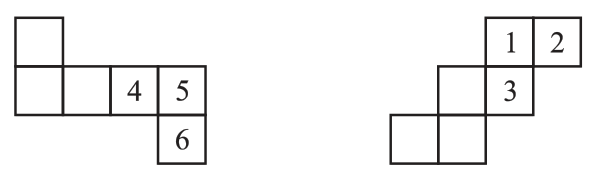Insert suitable numbers in the blanks, remembering that the number on the opposite faces should total to 7.3. Can this be a net for a die? Explain your answer.No, this cannot be a net for a die because one pair of opposite faces will have 1 and 4 on them and another pair of opposite faces will have 3 and 6 on them whose total is not equal to 7.

4. Here is an incomplete net for making a cube. Complete it in at least two different ways. Remember that a cube has six faces. How many faces are there in the net here? (Give two separate diagrams. If you like, you may use a squared sheet for easy manipulation.)There are three faces in the net.5. Match the nets with appropriate solids:(a) - (ii)
(b) - (iii)
(c) - (iv)
(d) - (i)

Exercise 15.2

1. Use isometric dot paper and make an isometric sketch for each one of the given shapes: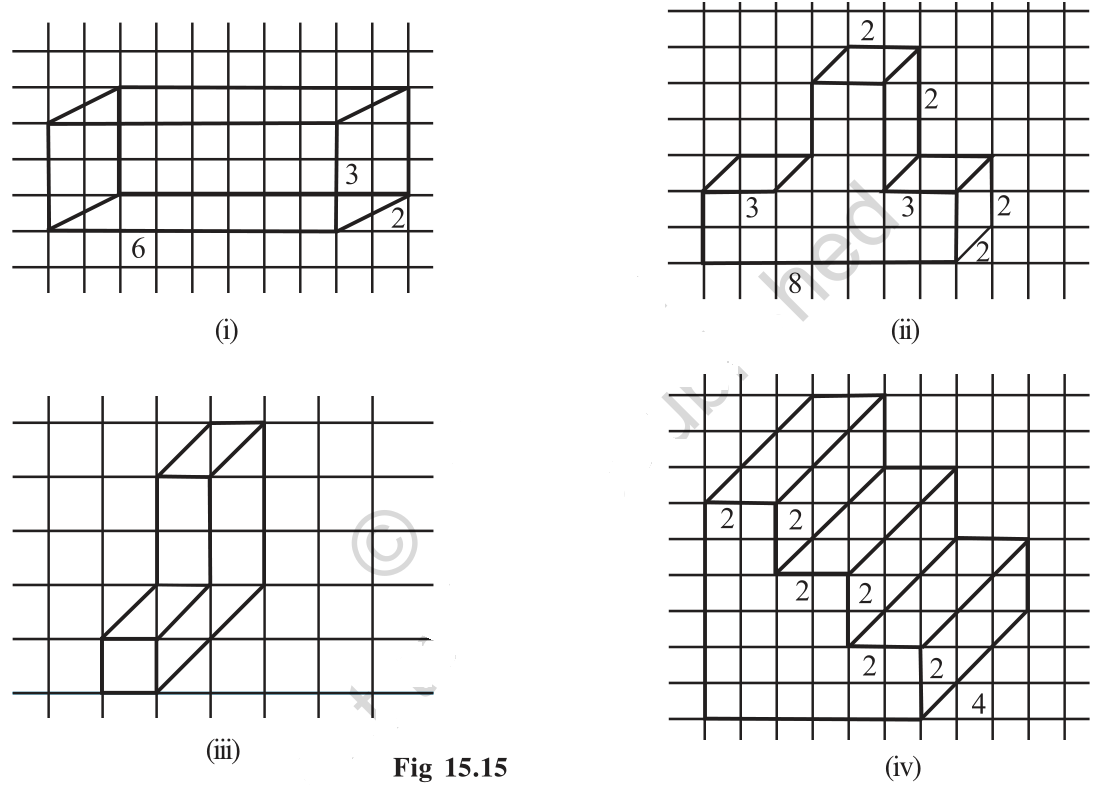2. The dimensions of a cuboid are 5 cm, 3 cm and 2 cm. Draw three different isometric sketches of this cuboid.

The dimensions of given cuboid are 5 cm, 3 cm and 2 cm:Three different isometric sketches are: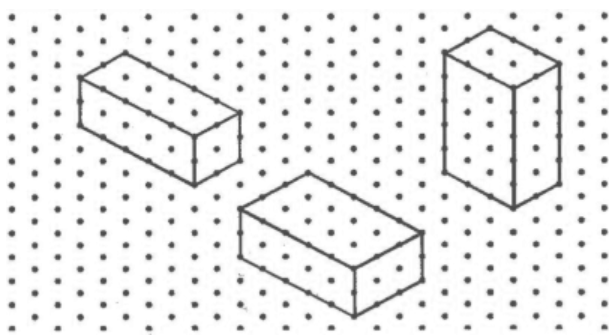3. Three cubes each with 2 cm edge are placed side by side to form a cuboid. Sketch an oblique or isometric sketch of this cuboid.

Oblique sketch: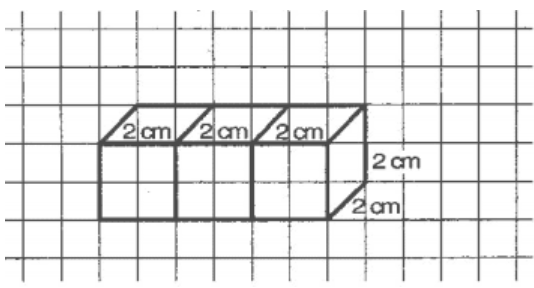Isometric sketch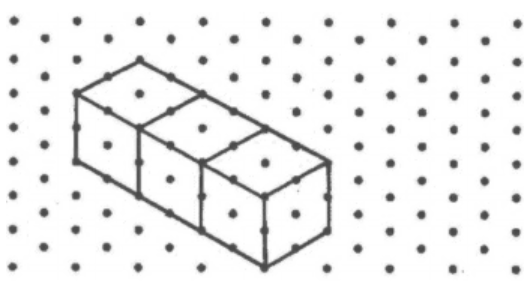4. Make an oblique sketch for each one of the given isometric shapes:5. Give
(i) an oblique sketch and (ii) an isometric sketch for each of the following:
(a) A cuboid of dimensions 5 cm, 3 cm and 2 cm. (Is your sketch unique?)
(b) A cube with an edge 4 cm long.

(a) A cuboid of dimensions 5 cm, 3 cm and 2 cm.

(i) Oblique sketch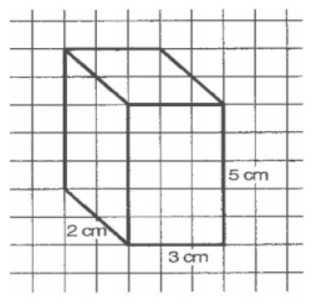(ii) Isometric sketch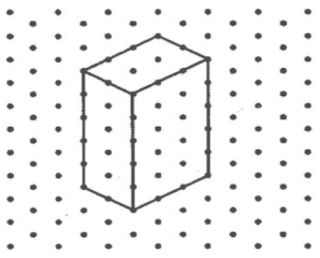(b) A cube with an edge 4 cm long.

(i) Oblique sketch(ii) Isometric sketch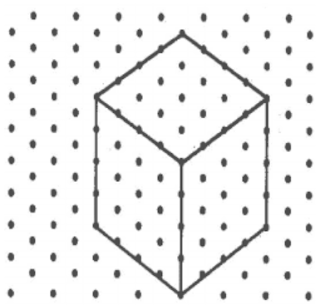6. An isometric sheet is attached at the end of the book. You could try to make on it some cubes or cuboids of dimensions specified by your friend.

Do it yourself.

Exercise 15.3

1. What cross-sections do you get when you give a:
(i) vertical cut (ii) horizontal cut to the following solids?
(a) A brick
(b) A round apple
(c) A die
(d) A circular pipe
(e) An ice-cream cone.

(i) Vertical cut:
(a) A brick – Cuboid
(b) A round apple - Circular
(c) A die – Square
(d) A circular pipe - Circular
(e) An ice cream cone – Curved shape

(ii) horizontal cut
(a) A brick - Cuboid
(b) A round apple - Circular
(c) A die - Cuboid
(d) A circular pipe - Hemispherical
(e) An ice cream cone - Frustum

Exercise 15.4

1. A bulb is kept burning just right above the following solids. Name the shape of the shadows obtained in each case. Attempt to give a rough sketch of the shadow.
(You may try to experiment first and then answer these questions).(i) A Ball - Circle
(ii) A cylindrical pipe - Line
(iii) A Book - Rectangle

2. Here are the shadows of some 3-D objects, when seen under the lamp of the overhead projector. Identify the solid (s) that match each shadow.
(There may be multiple answers for these!)(i) Circle - Chapatti, Football, Disc, Plate etc.
(ii) Square - Die, Square paper sheet, cubical magic box, Chalk box etc.
(iii) Triangle - Ice-cream cone, Birthday cap, etc.
(iv) Rectangle - Geometry box, Book, Table etc.

3. Examine if the following are true statements:
(i) The cube can cast a shadow in the shape of a rectangle.
(ii) The cube can cast a shadow in the shape of a hexagon.

(i) True
(ii) False.

Go Back To NCERT Solutions for Class 7 Maths

## NCERT Solutions for Class 7 Maths Chapter 15 Visualising Solid Shapes

Chapter 15 NCERT Solutions will encourage students to learn new topics and and get deeper understanding of various topics. By Visualizing solid shapes, we can see the hidden parts of a solid shape.

• All two-dimensional figures have only length and breadth.

• All three-dimensional solid shapes have length, breadth and height. The flat surfaces that form the skin of solids are called faces. The line segments that form the skeleton of solids are called edges. The points where three edges meet are called vertices.

• Solid shapes can be drawn on a flat surface, which is known as the two-dimensional representation of a three-dimensional solid.

If you're looking for specific questions then you can get exercisewise NCERT Solutions for Class 7 below which will enrich your knowledge and and getting command over questions. It will help in solving the difficulties that lie ahead with ease.

• Exercise 15.1 Chapter 15 Class 7 Maths NCERT Solutions
• Exercise 15.2 Chapter 15 Class 7 Maths NCERT Solutions
• Exercise 15.3 Chapter 15 Class 7 Maths NCERT Solutions
• Exercise 15.4 Chapter 15 Class 7 Maths NCERT Solutions

### NCERT Solutions for Class 7 Maths Chapters:

 Chapter 1 Integers Chapter 2 Fractions and Decimals Chapter 3 Data Handling Chapter 4 Simple Equations Chapter 5 Lines and Angles Chapter 6 The Triangle and its Properties Chapter 7 Congruence of Triangles Chapter 8 Comparing Quantities Chapter 9 Rational Numbers Chapter 10 Practical Geometry Chapter 11 Perimeter and Area Chapter 12 Algebraic Expressions Chapter 13 Exponents and Powers Chapter 14 Symmetry

FAQ on Chapter 15 Visualising Solid Shapes

#### How many exercises are there in Chapter 15 Visualising Solid Shapes Class 7 Maths NCERT Solutions?

There are 4 exercises in the Chapter 15 which will serve as beneficial tool that can be used to recall various questions any time. These Class 7 NCERT Solutions of Maths will help you in improving the marks in the examinations and have edge over your classmates.

#### What is the difference between Oblique and Isometric sketches?

Oblique sketches are drawn on squared paper. They do not have exact dimensions, but still convey all the significant aspects of the appearance of a solid while Isometric sketches are drawn on dotted or isometric sheets and have the exact measurements of solids.

#### What do you mean by nets for building 3d Shapes?

A net is a skeleton-out-line of a solid that can be folded to make it. The same solid can have several types of nets.

#### What cross-section do you get when you give a horizontal cut of Joker's cap?

We will get frustum by giving a horizontal cut of Joker's cap.